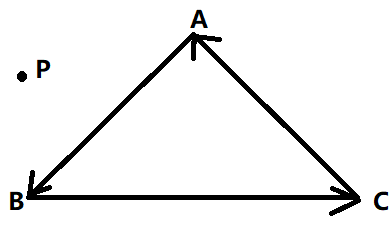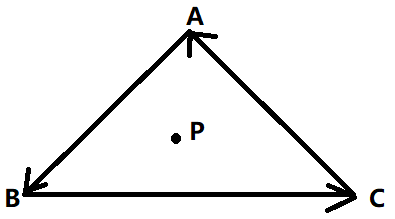# # 三角形 Trigonometry

## # 角度与弧度

### # 角度### # 弧度### # 换算

``````360º = 2π rad

1 rad =（180 /π）º ≈ 57.30º

α 度的角 =  α ·（π / 180）rad

``````
1
2
3
4
5
6
7
8## # 三角函数

### # 在直角三角形ABC中，∠C = 90º• 正弦（Sine）：sinA = ∠A的对边斜边的比
• 余弦（Cosine） ：cosA = ∠A的邻边斜边的比
• 正切（Tangent）：tanA = ∠A的对边邻边的比
• sin²A + cos²A = 1

### # 在单位圆（Unit Circle）中，任意角 α 的三角函数（Trigonometric Function）• 圆的圆心与直角坐标系的原点重合，圆的半径 r = 1，圆周上任意一点的坐标为（x, y）
• 自变量为角 α
• 函数是圆周上的坐标，或坐标和坐标的比值：sin α = y，cos α = x，tan α = y / x （x≠0）

### # 三角函数在各个象限的取值

sin α >0 >0 <0 <0 0 ±1
cos α >0 <0 <0 >0 ±1 0
tan α >0 <0 >0 <0 0 /

### # 正弦曲线• y = sin x， x∈R， y∈[–1，1]，周期为2π，函数图像以 x = (π/2) + kπ 为对称轴
• y = arcsin x， x∈[–1，1]， y∈[–π/2，π/2]
• sin x = 0 ←→ arcsin x = 0
• sin x = 1/2 ←→ arcsin x = π/6
• sin x = √2/2 ←→ arcsin x = π/4
• sin x = 1 ←→ arcsin x = π/2

### # 余弦曲线• y = cos x， x∈R， y∈[–1，1]，周期为2π，函数图像以 x = kπ 为对称轴
• y = arccos x， x∈[–1，1]， y∈[0，π]
• cos x = 0 ←→ arccos x = π/2
• cos x = 1/2 ←→ arccos x = π/3
• cos x = √2/2 ←→ arccos x = π/4
• cos x = 1 ←→ arccos x = 0

### # 三角函数线## # 一些公式

### # 平方### # 任意角α与-α的三角函数值之间的关系### # 两角和与差## # 常见问题

### # 一个点是否在三角形中？

• P点在△ABC外：• P点在△ABC内：``````static bool insideTriangle(int x, int y, const Vector3f* _v)
{
// Implement this function to check if the point (x, y)
// is inside the triangle represented by _v, _v, _v
Vector3f point(x + 0.5f, y + 0.5f, 1);
for (int i = 0; i < 3; i++)
{
Vector3f triangle2point = point - _v[i];
int index = (i + 1) % 3;
Vector3f triangleP2P = _v[index] - _v[i];
// case x , y cross equal z
if (triangleP2P.cross(triangle2point).z() < 0)
{
return false;
}
}

return true;
}
``````
1
2
3
4
5
6
7
8
9
10
11
12
13
14
15
16
17
18
19

### # 三角形重心1. 根据向量的线性相关：AP = βAB + γAC
2. 拆分上面式子：A - P = β(A - B) + γ(A - C)
3. 整理得到：P = (1-β-γ)A + βB + γC = αA + βB + γC
4. α = 1 - γ - β
5. 0 < α < 1, 0 < β < 1, 0 < γ < 1

• α = f12(x, y)/f12(x0, y0) ：点 PBC 线的距离与点 ABC 线的距离的比例
• β = f20(x, y)/f20(x1, y1) ：点 PCA 线的距离与点 BCA 线的距离的比例
• γ = f01(x, y)/f01(x2, y2) ：点 PAB 线的距离与点 CAB 线的距离的比例

• f01(x, y)=(y0 − y1)x + (x1 − x0)y + x0y1 − x1y0,
• f12(x, y)=(y1 − y2)x + (x2 − x1)y + x1y2 − x2y1,
• f20(x, y)=(y2 − y0)x + (x0 − x2)y + x2y0 − x0y2.

``````static std::tuple<float, float, float> computeBarycentric2D(float x, float y, const Vector4f *v)
{
float c1 = (x * (v.y() - v.y()) + (v.x() - v.x()) * y + v.x() * v.y() - v.x() * v.y()) / (v.x() * (v.y() - v.y()) + (v.x() - v.x()) * v.y() + v.x() * v.y() - v.x() * v.y());
float c2 = (x * (v.y() - v.y()) + (v.x() - v.x()) * y + v.x() * v.y() - v.x() * v.y()) / (v.x() * (v.y() - v.y()) + (v.x() - v.x()) * v.y() + v.x() * v.y() - v.x() * v.y());
float c3 = (x * (v.y() - v.y()) + (v.x() - v.x()) * y + v.x() * v.y() - v.x() * v.y()) / (v.x() * (v.y() - v.y()) + (v.x() - v.x()) * v.y() + v.x() * v.y() - v.x() * v.y());
return {c1, c2, c3};
}
``````
1
2
3
4
5
6
7